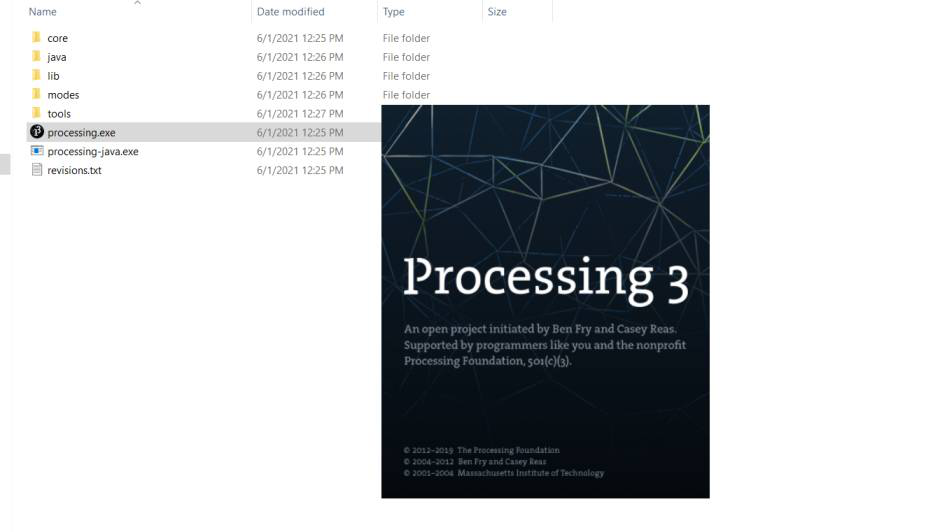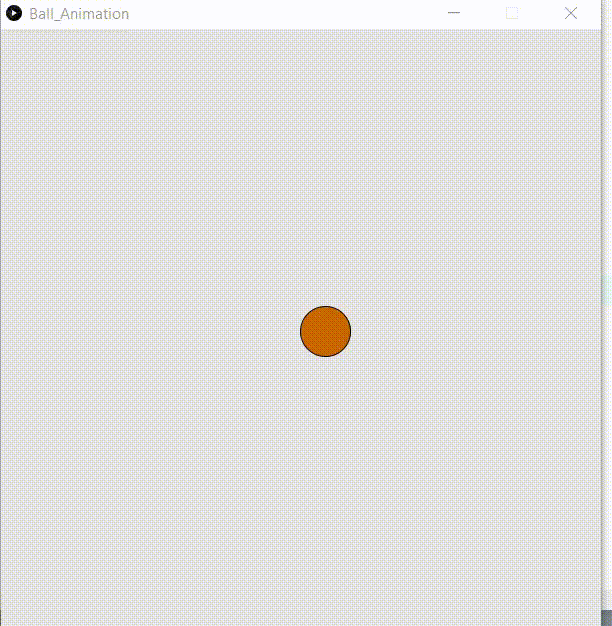# How to Set Up Processing on Windows?

• Last Updated : 19 Oct, 2021

Processing is Open Source Software that is used for the Electronic Arts and visual design communities. We can create different types of arts using our coding skills like games, animation And physics engine, etc.

To set up processing follow the below steps:Step 2: Extract The Zip file in any folder and open processing .exe.Step 3: The Processing IDE will be open where you can write your code.Example :

## Java

 `// Program to show moving Ball.` `// Set up variable position,colour and velocity.``PVector pos;``PVector vel;``int` `col;` `// Function to set up size of canvas``// and position,velocity and colour.``void` `setup(){``  ``size(``600``, ``600``);``  ``pos = ``new` `PVector(width/``2``, height/``2``);``  ``vel = ``new` `PVector(random(-``4``, ``4``), random(-``4``, ``4``));``  ``col = floor(random(``0``, ``255``));``}` `// Function to draw eclipse.``void` `draw(){``  ``background(col);``  ``fill(``231``);``  ``checkValid();``  ``fill(``204``, ``102``, ``0``);``  ``ellipse(pos.x, pos.y, ``50``, ``50``);``  ``pos.add(vel);``}` `// Function to check the position``// of ball must be wthin screen.``void` `checkValid(){``  ``if``(pos.x <= ``25` `|| pos.x >= width - ``25``){``    ``vel.x *= -``1``;``    ` `    ``// Change in colour when it hit the wall.``    ``col = floor(random(``0` `,``255``));``  ``}``  ``if``(pos.y <=``25` `|| pos.y >= height - ``25``){``    ``vel.y *= -``1``;``    ` `    ``// Change in colour when it hit the wall.``    ``col = floor(random(``0` `,``255``));``  ``}``  ` `}`

Output:My Personal Notes arrow_drop_up